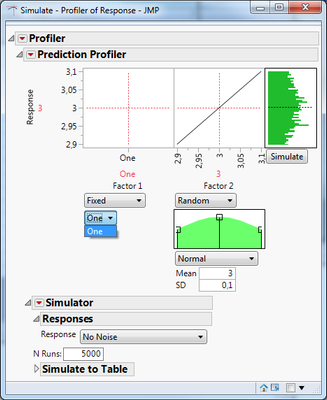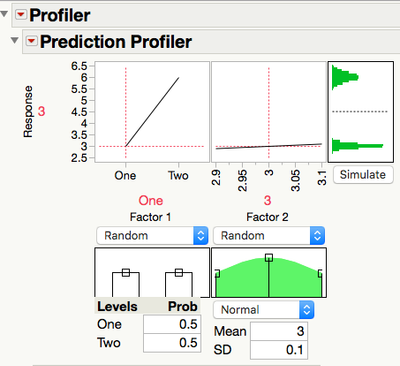Choose Language Hide Translation Bar
Highlighted

## Nominal factor in Prediction Profiler

Dear community,

I am trying to use the prediction profiler with one of the factors having the nominal data type.  In the example below, the Factor 1 can assume two values, “One” and “Two”. The formula to be profiled contains a ‘Match’ statement that selects the numerical value 1 or 2, according to which string (“One” or “Two”) is entered, see the example code:

dt = New Table("Simulate",

New Column("Factor 1",

Character,

"Nominal",

Set Values({"One"})

),

New Column("Factor 2",

Numeric,

"Continuous",

Format("Best", 12),

Set Values()

),

New Column("Response",

Numeric,

"Continuous",

Format("Best", 12),

Formula(Match( :Factor 1, "One", 1, "Two", 2 ) * :Factor 2)

)

);

Profiler(

Y(:Response),

Simulator(

Factors(

Factor 2 << Random( Normal( 3, 0.1 ) )

)

)

)

When I run this code, a profiler report opens, like the one shown.Now, here is my question: How do I get the dropdown menu below the graph for Factor 1 to show both options “One” and “Two”? The profiler and the simulation result should then change, according to which string value is chosen for Factor 1.

Any help is much appreciated.

6 REPLIES 6
Highlighted

## Re: Nominal factor in Prediction Profiler

dt = New Table("Simulate",

New Column("Factor 1",

Character,

"Nominal",

Set Values({"One", "Two"})

),

New Column("Factor 2",

Numeric,

"Continuous",

Format("Best", 12),

Set Values([3, 3])

),

New Column("Response",

Numeric,

"Continuous",

Format("Best", 12),

Formula(Match( :Factor 1, "One", 1, "Two", 2 ) * :Factor 2)

)

);

Profiler(

Y(:Response),

Simulator(

Factors(

Factor 2 << Random( Normal( 3, 0.1 ) )

)

)

);Highlighted

## Re: Nominal factor in Prediction Profiler

Thank you for your reply. Let me just elaborate on what I want to do. I am simulating the heat transmission through a window pane which is given by an analytical expression. One of the parameters determining the heat flow is the type of material used for the glazing. I want the user to be able to select the type of material (corresponding to "One" and "Two" above), and have one distribution as the simulation output. Choosing the material corresponds to setting the material specific constants in the prediction formula. It is not a  matter of assigning a probability for which material the pane might be made of, it is simply a question of defining a constant in the prediction formula as a result of a user input. Using your suggested solution I could just set all probabilities to 0 except for the material I want to use. I was just wondering if there is a way to achieve this without having to adjust the probabilities for each possible material (there might be more than just two). Also, a mixture of probabilities does not really make any sense for my application.

Highlighted

## Re: Nominal factor in Prediction Profiler

Sorry, I may have misled you. Setting 'Factor 1' to 'Random' was for illustration only - If you keep it fixed you can then select which level you keep it fixed at.

Highlighted

## Re: Nominal factor in Prediction Profiler

Perfect! Sometimes the answer is easy... One last thing. Is it possible to 'link' the choice of string value for Factor 1 to the value marked by the red cross in the graph? The 'link' seems to work one way only. Moving the red cross sets the value in the drop down, but not the other way around.

HighlightedJeff_PerkinsonCommunity Manager

## Re: Nominal factor in Prediction Profiler

Looks like you've found a bug with respect to the factor setting in the graph and drop down not always moving together. I've reported that and we'll see if we can get it addressed in a future release.

-Jeff

-Jeff
Highlighted

## Re: Nominal factor in Prediction Profiler

When I define a factor as random and use a non-normal distribution there seems to be no link at all.

Article Labels

There are no labels assigned to this post.